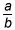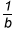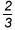# Activities

••• ##### Subject Area

• Math: Elementary Math: Fractions

• ##### AuthorK-5

30 Minutes

• ##### Device
•TI-Nspire™ CX/CX II
•TI-Nspire™ CX CAS/CX II CAS
• ##### Software

TI-Nspire™
TI-Nspire™ CAS

## Multiplying Fractions by a Whole Number

#### Activity Overview

This activity uses a number line to develop multiplication of a fraction by a whole number. It builds on the earlier concept that the product of two whole numbers r and s is r sets, each containing s elements.

This TI-Nspire™ lesson uses a number line to develop multiplication of a fraction by a whole number. It builds on the earlier concept that the product of two whole numbers r and s is r sets, each containing s elements. Using the same reasoning in the context of a number line, the product of c and the fractionwould be c copies of, which is equivalent to ca copies of. Students learn how to mark a “unit” such ason a number line (e.g., a product is sold in units ofof a pound) and visualize the value of 5 copies of(e.g., the amount you would have with five units).